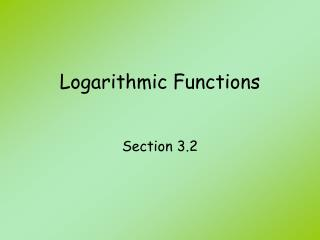DownloadDownload PresentationLogarithmic Functions

# Logarithmic Functions

Télécharger la présentation## Logarithmic Functions

- - - - - - - - - - - - - - - - - - - - - - - - - - - E N D - - - - - - - - - - - - - - - - - - - - - - - - - - -
##### Presentation Transcript

1. Logarithmic Functions Section 3.2

2. Objectives • Rewrite an exponential equation in logarithmic form. • Rewrite a logarithmic equation in exponential form. • Evaluate a simple logarithmic expression. • Find a missing piece of a logarithmic equation given all the other pieces. • Find the equation of a logarithmic function given a point. • Graph a logarithmic function. • Determine the transformation of a logarithmic function. • State the domain and range of a logarithmic function.

3. Vocabulary • logarithmic function • base of a logarithmic function • logarithmic form • exponential form • common logarithm • natural logarithm

4. Find the inverse of the function f:

5. Formula • basic logarithmic function where a > 0 and a≠ 1

6. Express each equation in logarithmic form.

7. Express each equation in exponential form.

8. Graph the function What it the domain of the function? What is the range of the function? What is(are) the x-intercepts? What is(are) the y-intercepts?

9. Evaluate the following

10. Evaluate the following

11. Solve for x.

12. Find the domain and range of

13. Properties of Logarithms